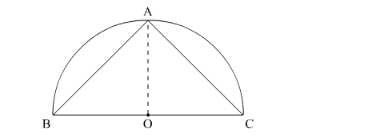# The area of the largest triangle that can be inscribed

Question:

The area of the largest triangle that can be inscribed in a semi-circle of radius r, is

(a) $r^{2}$

(b) $2 r^{2}$

(c) $r^{3}$

(d) $2 r^{3}$

Solution:

The triangle with the largest area will be symmetrical as shown in the figure.Let the radius of the circle be r.

Hence,

$a r(\Delta \mathrm{ABC})=\frac{1}{2}(r)(2 r)$

$=r^{2} s q$, unit